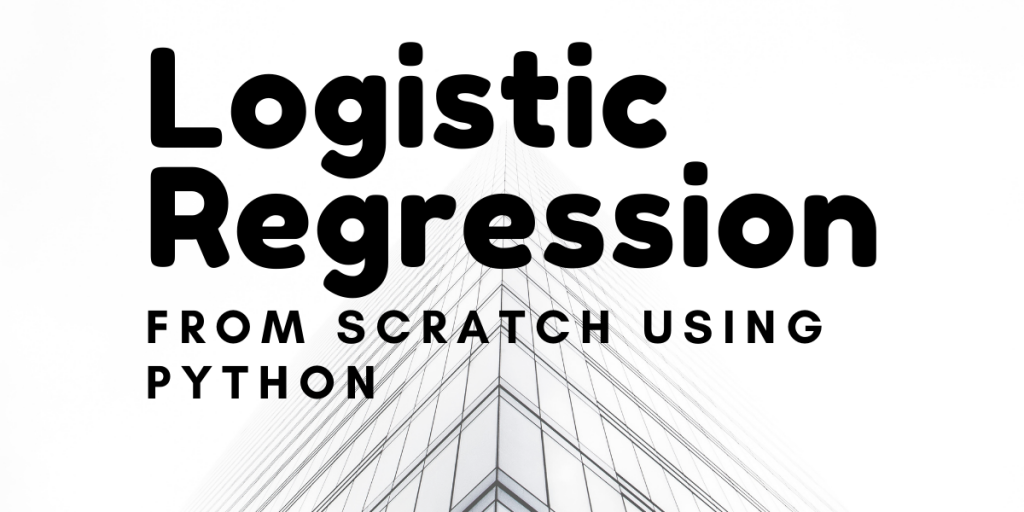# Logistic Regression From Scratch in Python [Algorithm Explained]The objective of this tutorial is to implement our own Logistic Regression from scratch. This is going to be different from our previous tutorial on the same topic where we used built-in methods to create the function.

Logistic regression is a classic method mainly used for Binary Classification problems. even though it can be used for multi-class classification problems with some modification, in this article we will perform binary classification.

## Implementing Logistic Regression from Scratch

Step by step we will break down the algorithm to understand its inner working and finally will create our own class.

### Step-1: Understanding the Sigmoid function

The sigmoid function in logistic regression returns a probability value that can then be mapped to two or more discrete classes. Given the set of input variables, our goal is to assign that data point to a category (either 1 or 0). The sigmoid function outputs the probability of the input points belonging to one of the classes.

```#Defining a sigmoid function
def sigmoid(z):
op = 1/(1 + np.exp(-z))
return op
```

### Step-2: The Loss Function

The loss function consists of parameters/weights, when we say we want to optimize a loss function by this we simply refer to finding the best values of the parameters/weights.

The loss function for Logistic Regression is defined as:

```#Loss Function

def loss(h, y):
return (-y * np.log(h) - (1 - y) * np.log(1 - h)).mean()
```

The Gradient descent is just the derivative of the loss function with respect to its weights.

We get this after we find find the derivative of the loss function:

```#Gradient_descent

return np.dot(X.T, (h - y)) / y.shape
```

The weights are updated by subtracting the derivative (gradient descent) times the learning rate. Updating the weights:

Here – alpha is the learning rate.

## Putting it all together

Let’s create a class to compile the steps mentioned above. Here’s the complete code for implementing Logistic Regression from scratch. We have worked with the Python numpy module for this implementation.

```#import required modules
import numpy as np

class LogisticRegression:
def __init__(self,x,y):
self.intercept = np.ones((x.shape, 1))
self.x = np.concatenate((self.intercept, x), axis=1)
self.weight = np.zeros(self.x.shape)
self.y = y

#Sigmoid method
def sigmoid(self, x, weight):
z = np.dot(x, weight)
return 1 / (1 + np.exp(-z))

#method to calculate the Loss
def loss(self, h, y):
return (-y * np.log(h) - (1 - y) * np.log(1 - h)).mean()

return np.dot(X.T, (h - y)) / y.shape

def fit(self, lr , iterations):
for i in range(iterations):
sigma = self.sigmoid(self.x, self.weight)

loss = self.loss(sigma,self.y)

dW = self.gradient_descent(self.x , sigma, self.y)

#Updating the weights
self.weight -= lr * dW

return print('fitted successfully to data')

#Method to predict the class label.
def predict(self, x_new , treshold):
x_new = np.concatenate((self.intercept, x_new), axis=1)
result = self.sigmoid(x_new, self.weight)
result = result >= treshold
y_pred = np.zeros(result.shape)
for i in range(len(y_pred)):
if result[i] == True:
y_pred[i] = 1
else:
continue

return y_pred

```

To implement the Algorithm we defined a `fit` method which requires the learning rate and the number of iterations as the input arguments.

The above class can be initialized by providing the input data and the target values.

### Now, it’s time to test our implementation.

```from sklearn.datasets import load_breast_cancer

#Preparing the data
x = data.data
y = data.target

#creating the class Object
regressor = LogisticRegression(x,y)

#
regressor.fit(0.1 , 5000)

y_pred = regressor.predict(x,0.5)

print('accuracy -> {}'.format(sum(y_pred == y) / y.shape))
```

Output:

```fitted successfully to data
accuracy -> 0.9209138840070299
```

Our implemented model achieved accuracy of 92%, not bad.

You can find the notebook for this tutorial here on my GitHub Repository.

## Conclusion

This article was all about implementing a Logistic Regression Model from scratch to perform a binary classification task. We also unfold the inner working of the regression algorithm by coding it from 0.

Till we meet next time. Happy Learning!